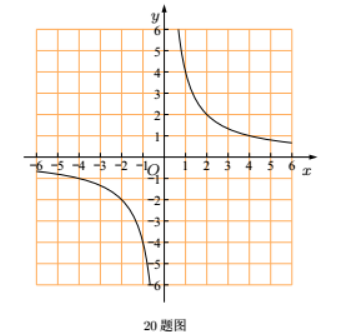(1) 求一次函数的表达式, 并在图中画出这个一次函数的图象:
(2) 根据函数图象, 直接写出不等式 $k x+b > \frac{4}{x}$ 的解集:
(3) 若点 $C$ 是点 $B$ 关于 $y$ 轴的对称点, 连接 $A C, B C$, 求 $\triangle A B C$ 的面积.①点击 首页查看更多试卷和试题 , 点击查看 本题所在试卷